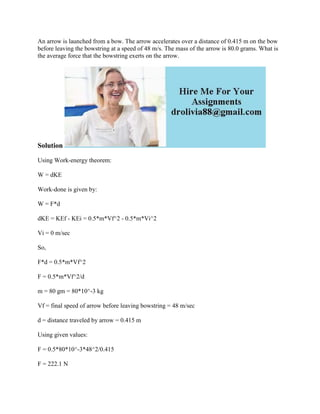Se está descargando tu SlideShare. ×

# An arrow is launched from a bow- The arrow accelerates over a distance.docx

Anuncio
Anuncio
Anuncio
Anuncio
Anuncio
Anuncio
Anuncio
Anuncio
Anuncio
Anuncio
AnuncioCargando en…3
×

1 de 2 Anuncio

# An arrow is launched from a bow- The arrow accelerates over a distance.docx

An arrow is launched from a bow. The arrow accelerates over a distance of 0.415 m on the bow before leaving the bowstring at a speed of 48 m/s. The mass of the arrow is 80.0 grams. What is the average force that the bowstring exerts on the arrow.
Solution
Using Work-energy theorem:
W = dKE
Work-done is given by:
W = F*d
dKE = KEf - KEi = 0.5*m*Vf^2 - 0.5*m*Vi^2
Vi = 0 m/sec
So,
F*d = 0.5*m*Vf^2
F = 0.5*m*Vf^2/d
m = 80 gm = 80*10^-3 kg
Vf = final speed of arrow before leaving bowstring = 48 m/sec
d = distance traveled by arrow = 0.415 m
Using given values:
F = 0.5*80*10^-3*48^2/0.415
F = 222.1 N
.

An arrow is launched from a bow. The arrow accelerates over a distance of 0.415 m on the bow before leaving the bowstring at a speed of 48 m/s. The mass of the arrow is 80.0 grams. What is the average force that the bowstring exerts on the arrow.
Solution
Using Work-energy theorem:
W = dKE
Work-done is given by:
W = F*d
dKE = KEf - KEi = 0.5*m*Vf^2 - 0.5*m*Vi^2
Vi = 0 m/sec
So,
F*d = 0.5*m*Vf^2
F = 0.5*m*Vf^2/d
m = 80 gm = 80*10^-3 kg
Vf = final speed of arrow before leaving bowstring = 48 m/sec
d = distance traveled by arrow = 0.415 m
Using given values:
F = 0.5*80*10^-3*48^2/0.415
F = 222.1 N